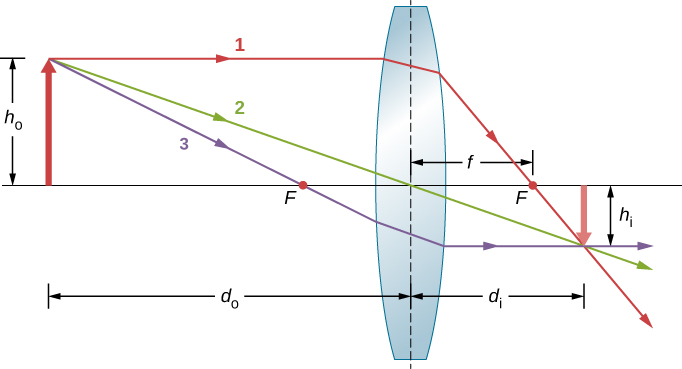# 2.4 Thin lenses  (Page 3/13)

 Page 3 / 13

## Image formation by thin lenses

We use ray tracing to investigate different types of images that can be created by a lens. In some circumstances, a lens forms a real image, such as when a movie projector casts an image onto a screen. In other cases, the image is a virtual image, which cannot be projected onto a screen. Where, for example, is the image formed by eyeglasses? We use ray tracing for thin lenses to illustrate how they form images, and then we develop equations to analyze quantitatively the properties of thin lenses.

Consider an object some distance away from a converging lens, as shown in [link] . To find the location and size of the image, we trace the paths of selected light rays originating from one point on the object, in this case, the tip of the arrow. The figure shows three rays from many rays that emanate from the tip of the arrow. These three rays can be traced by using the ray-tracing rules given above.

• Ray 1 enters the lens parallel to the optical axis and passes through the focal point on the opposite side (rule 1).
• Ray 2 passes through the center of the lens and is not deviated (rule 2).
• Ray 3 passes through the focal point on its way to the lens and exits the lens parallel to the optical axis (rule 3).

The three rays cross at a single point on the opposite side of the lens. Thus, the image of the tip of the arrow is located at this point. All rays that come from the tip of the arrow and enter the lens are refracted and cross at the point shown.

After locating the image of the tip of the arrow, we need another point of the image to orient the entire image of the arrow. We chose to locate the image base of the arrow, which is on the optical axis. As explained in the section on spherical mirrors, the base will be on the optical axis just above the image of the tip of the arrow (due to the top-bottom symmetry of the lens). Thus, the image spans the optical axis to the (negative) height shown. Rays from another point on the arrow, such as the middle of the arrow, cross at another common point, thus filling in the rest of the image.

Although three rays are traced in this figure, only two are necessary to locate a point of the image. It is best to trace rays for which there are simple ray-tracing rules.Ray tracing is used to locate the image formed by a lens. Rays originating from the same point on the object are traced—the three chosen rays each follow one of the rules for ray tracing, so that their paths are easy to determine. The image is located at the point where the rays cross. In this case, a real image—one that can be projected on a screen—is formed.

Several important distances appear in the figure. As for a mirror, we define ${d}_{\text{o}}$ to be the object distance, or the distance of an object from the center of a lens. The image distance ${d}_{\text{i}}$ is defined to be the distance of the image from the center of a lens. The height of the object and the height of the image are indicated by ${h}_{\text{o}}$ and ${h}_{\text{i}}$ , respectively. Images that appear upright relative to the object have positive heights, and those that are inverted have negative heights. By using the rules of ray tracing and making a scale drawing with paper and pencil, like that in [link] , we can accurately describe the location and size of an image. But the real benefit of ray tracing is in visualizing how images are formed in a variety of situations.

What is the importance of de-broglie's wavelength?
he related wave to matter
Zahid
at subatomic level wave and matter are associated. this refering to mass energy equivalence
Zahid
how those weight effect a stable motion at equilibrium
how do I differentiate this equation- A sinwt with respect to t
just use the chain rule : let u =wt , the dy/dt = dy/du × du/dt : wA × cos(wt)
Jerry
I see my message got garbled , anyway use the chain rule with u= wt , etc...
Jerry
de broglie wave equation
vy beautiful equation
chandrasekhar
what is electro statics
when you consider systems consisting of fixed charges
Sherly
Diagram of the derive rotational analog equation of v= u+at
what is carat
a unit of weight for precious stones and pearls, now equivalent to 200 milligrams.
LoNE
a science that deals with the composition, structure, and properties of substances and with the transformations that they undergo.
LoNE
what is chemistry
what chemistry ?
Abakar
where are the mcq
ok
Giorgi
acids and bases
Navya
How does unpolarized light have electric vector randomly oriented in all directions.
unpolarized light refers to a wave collection which has an equal distribution of electric field orientations for all directions
pro
In a grating, the angle of diffraction for second order maximum is 30°.When light of wavelength 5*10^-10cm is used. Calculate the number of lines per cm of the grating.
OK I can solve that for you using Bragg's equation 2dsin0over lander
ossy
state the law of gravity 6
what is cathodic protection
its just a technique used for the protection of a metal from corrosion by making it cathode of an electrochemical cell.
akif
what is interferometer
what is interferometer
Abakar
Show that n1Sino1=n2Sino2ByBy Sebastian Sieczko...ByBy